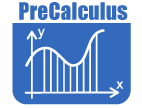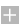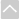# PreCalculusResources by Strand

Strand: MATHEMATICAL PRACTICES (MP)
The Standards for Mathematical Practice in PreCalculus describe mathematical habits of mind that teachers should seek to develop in their students. Students become mathematically proficient in engaging with mathematical content and concepts as they learn, experience, and apply these skills and attitudes (Standards MP.1-8).Strand: NUMBER AND QUANTITY - Vector and Matrix Quantities (N.VM)
Represent and model with vector quantities (Standards 1–3).

Standard N.VM.1
Recognize vector quantities as having both magnitude and direction. Represent vector quantities by directed line segments, and use appropriate symbols for vectors and their magnitudes (e.g., v, |v|, ||v||, v).

Standard N.VM.2
Find the components of a vector by subtracting the coordinates of an initial point from the coordinates of a terminal point.

Standard N.VM.3
Solve problems involving velocity and other quantities that can be represented by vectors.

Perform operations on vectors (Standards 4–5).

Standard N.VM.4

1. Add vectors end to end, component-wise, and by the parallelogram rule. Understand that the magnitude of a sum of two vectors is typically not the sum of the magnitudes.
2. Given two vectors in magnitude and direction form, determine the magnitude and direction of their sum.
3. Understand vector subtraction v - w as v + (-w), where -w is the additive inverse of w, with the same magnitude as w and pointing in the opposite direction. Represent vector subtraction graphically by connecting the tips in the appropriate order, and perform vector subtraction component-wise.

Standard N.VM.5
Multiply a vector by a scalar.

1. Represent scalar multiplication graphically by scaling vectors and possibly reversing their direction; perform scalar multiplication component-wise, e.g., as c(vx , vy ) = (cvx, cry ).
2. Compute the magnitude of a scalar multiple cv using ||cv|| = |c|v. Compute the direction of cv knowing that when |c|v0, the direction of cv is either along v (for c > 0) or against vs (for c < 0).
Perform operations on matrices and use matrices in applications (Standards 6–13).

Standard N.VM.6
Use matrices to represent and manipulate data, e.g., to represent payoffs or incidence relationships in a network.

Standard N.VM.7
Multiply matrices by scalars to produce new matrices, e.g., as when all of the payoffs in a game are doubled.

Standard N.VM.8
Add, subtract, and multiply matrices of appropriate dimensions.

Standard N.VM.9
Understand that, unlike multiplication of numbers, matrix multiplication for square matrices is not a commutative operation, but still satisfies the associative and distributive properties.

Standard N.VM.10
Understand that the zero and identity matrices play a role in matrix addition and multiplication similar to the role of 0 and 1 in the real numbers. The determinant of a square matrix is nonzero if and only if the matrix has a multiplicative inverse.

Standard N.VM.11
Multiply a vector (regarded as a matrix with one column) by a matrix of suitable dimensions to produce another vector. Work with matrices as transformations of vectors.

Standard N.VM.12
Work with 2 × 2 matrices as transformations of the plane, and interpret the absolute value of the determinant in terms of area.

Standard N.VM.13
Solve systems of linear equations up to three variables using matrix row reduction.Strand: NUMBER AND QUANTITY - Complex Number Systems (N.CN)
Perform arithmetic operations with complex numbers (Standard 3)

Standard N.CN.3
Find the conjugate of a complex number; use conjugates to find moduli and quotients of complex numbers.

Represent complex numbers and their operations on the complex plane (Standards 4–6).

Standard N.CN.4
Represent complex numbers on the complex plane in rectangular and polar form (including real and imaginary numbers), and explain why the rectangular and polar forms of a given complex number represent the same number.

Standard N.CN.5
Represent addition, subtraction, multiplication, and conjugation of complex numbers geometrically on the complex plane; use properties of this representation for computation. For example, (-1 + √3 i)3 = 8, because (-1 + √3 i) has modulus 2 and argument 120°.

Standard N.CN.6
Calculate the distance between numbers in the complex plane as the modulus of the difference, and the midpoint of a segment as the average of the numbers at its endpoints.

Use complex numbers in polynomial identities and equations (Standard 10).

Standard N.CN.10
Multiply complex numbers in polar form and use DeMoivre's Theorem to find roots of complex numbers.Strand: ALGEBRA: Reasoning With Equations and Inequalities (A.REI)
Solve systems of equations (Standards 8–9).

Standard A.REI.8
Represent a system of linear equations as a single matrix equation in a vector variable.

Standard A.REI.9
Find the inverse of a matrix, if it exists, and use it to solve systems of linear equations (using technology for matrices of dimension 3 x 3 or greater).Strand: FUNCTIONS - Interpreting Functions (F.IF)
Analyze functions using different representations (Standard 7, 10–11).

Standard F.IF.7
Graph functions expressed symbolically, and show key features of the graph, by hand in simple cases and using technology for more complicated cases.

1. Graph rational functions, identifying zeros, asymptotes, and point discontinuities when suitable factorizations are available, and showing end behavior.
2. Define a curve parametrically and draw its graph.

Standard F.IF.10
Use sigma notation to represent the sum of a finite arithmetic or geometric series.

Standard F.IF.11
Represent series algebraically, graphically, and numerically.Strand: FUNCTIONS - Building Functions (F.BF)
Build a function that models a relationship between two quantities (Standard 1)

Standard F.BF.1
Write a function that describes a relationship between two quantities.

1. Compose functions. For example, if T(y) is the temperature in the atmosphere as a function of height, and h(t) is the height of a weather balloon as a function of time, then T(h(t)) is the temperature at the location of the weather balloon as a function of time.
Build new functions from existing functions (Standard 4–5).

Standard F.BF.4
Find inverse functions.

1. Verify by composition that one function is the inverse of another.
2. Read values of an inverse function from a graph or a table, given that the function has an inverse.
3. Produce an invertible function from a non-invertible function by restricting the domain.

Standard F.BF.5
Understand the inverse relationship between exponents and logarithms and use this relationship to solve problems involving logarithms and exponents.Strand: FUNCTIONS - Trigonometric Functions (F.TF)
Extend the domain of trigonometric functions using the unit circle (Standard 4)

Standard F.TF.4
Use the unit circle to explain symmetry (odd and even) and periodicity of trigonometric functions.

Model periodic phenomena with trigonometric functions (Standard 6–7).

Standard F.TF.6
Understand that restricting a trigonometric function to a domain on which it is always increasing or always decreasing allows its inverse to be constructed.

Standard F.TF.7
Use inverse functions to solve trigonometric equations that arise in modeling contexts; evaluate the solutions using technology, and interpret them in terms of the context

Prove and apply trigonometric identities (Standard 9).

Standard F.TF.9
Prove the addition and subtraction formulas for sine, cosine, and tangent, and use them to solve problems.Strand: GEOMETRY - Geometric Measurement and Dimension (G.GMD)
Explain volume formulas and use them to solve problems (Standard 2).

Standard G.GMD.2
Give an informal argument using Cavalieri's principle for the formulas for the volume of a sphere and other solid figures.Strand: GEOMETRY - Expressing Geometric Properties With Equations (G.GPE)
Translate between the geometric description and the equation for a conic section (Standards 2–3).

Standard G.GPE.2
Derive the equation of a parabola given a focus and a directrix.

Standard G.GPE.3
Derive the equations of ellipses and hyperbolas given the foci, using the fact that the sum or difference of distances from the foci is constant.Strand: STATISTICS - Conditional Probability and the Rules of Probability (S.CP)
Understand independence and conditional probability and use them to interpret data (Standards 2–3).

Standard S.CP.2
Understand that two events A and B are independent if the probability of A and B occurring together is the product of their probabilities, and use this characterization to determine if they are independent.

Standard S.CP.3
Understand the conditional probability of A given B as P(A and B)/P(B), and interpret independence of A and B as saying that the conditional probability of B given A is the same as the probability of B.

Use the rules of probability to compute probabilities of compound events in a uniform probability model (Standards 7–9).

Standard S.CP.7
Apply the Addition Rule, P(A or B) = P(A) + P(B) - P(A and B), and interpret the answer in terms of the model.

Standard S.CP.8
Apply the general Multiplication Rule in a uniform probability model, P(A andB) = P(A)P(B|A) = P(B)P(A|B), and interpret the answer in terms of the model.

Standard S.CP.9
Use permutations and combinations to compute probabilities of compound events and solve problems.The Online Core Resource pages are a collaborative project between the Utah State Board of Education and the Utah Education Network. If you would like to recommend a high quality resource, contact Trish French (Elementary) or Lindsey Henderson (Secondary). If you find inaccuracies or broken links contact resources@uen.org.